Function Repository Resource:

# CarlemanMatrix

Evaluate the Carleman matrix of a function

Contributed by: Jan Mangaldan
 ResourceFunction["CarlemanMatrix"][f,{x,x0,{m,n}}] gives the order {m,n} Carleman matrix of f about the point x=x0. ResourceFunction["CarlemanMatrix"][f,{x,x0,n}] gives the order n Carleman matrix of f about the point x=x0.

## Details

The Carleman matrix of an infinitely differentiable function f(x) has elements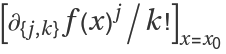.
The Carleman matrix about the point x=x0 is defined only when there is a corresponding power series at that point.
The order {m,n} Carleman matrix has dimensions (m+1)×(n+1).

## Examples

### Basic Examples (1)

A Carleman matrix of order {2,3} for Exp[x]:

 In:=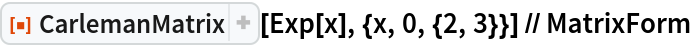Out=### Scope (2)

Carleman matrix of an arbitrary function:

 In:=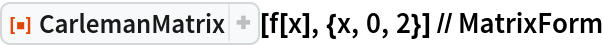Out=Carleman matrix with a complex-valued expansion point:

 In:=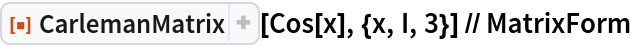Out=### Properties and Relations (3)

The kth row of the Carleman matrix of f(x) corresponds to the power series coefficients of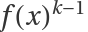:

 In:=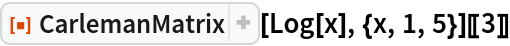Out=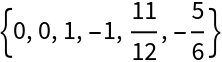In:=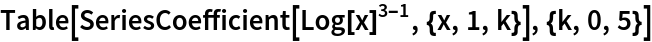Out=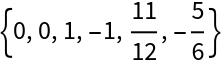If f(0)=0, the second row of the nth matrix power of the Carleman matrix of f(x) corresponds to the power series coefficients of the nth composition of f(x):

 In:=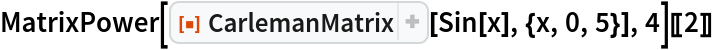Out=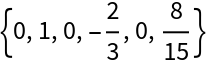In:=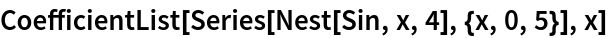Out=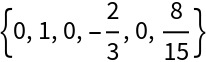If f(0)=0, the second row of the inverse of the Carleman matrix of f(x) corresponds to the power series coefficients of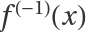:

 In:=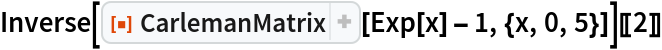Out=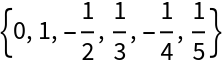In:=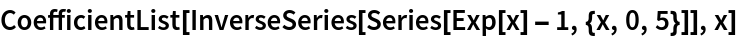Out=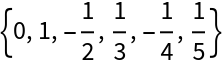## Version History

• 1.0.0 – 04 March 2021